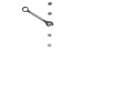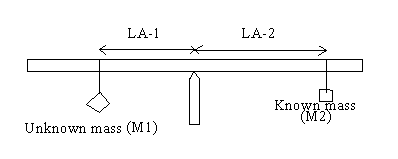# Torque

When a force produces rotation, the result is called a torque. Torque is the product of the force applied to an object times the perpendicular distance from the applied force to the center of the axis of rotation. τ = r X F sin θ

Torque is measured in N-m in the SI system.

Torque is a measure of the magnitude of the tendency of a force to rotate an object about some axis. You can loosely think of torque as the rotational/circular equivalent of force.

torque = Fd

(The symbol for torque is the Greek letter tau, which is.)

….where d is the distance of the lever arm (or moment arm); the lever arm is the perpendicular distance from the axis of rotation to a line drawn along the direction of the force. The value of the torque depends on the axis of rotation. (see below)In the drawing above, torque = FL sin θ

The sign of the torque (a vector quantity) is positive if its turning tendency is counterclockwise and negative if its turning tendency is clockwise. (Like measuring angles from east and going towards north is positive angles and therefore CCW, from east and going towards south is a negative angle and therefore CW.)

The sum of the torques acting on any object is

Σ τ =1 +2 +3 ….

As with work, torque is measured in a combination of force and distance units: N-m or ft-lb.

When the sum of the forces acting on an object is 0, the object is in equilibrium (not necessarily at rest, just not changing…so therefore can be v=0 or v= a constant and zero acceleration).

First condition of equilibrium Σ F= 0 (translational equilibrium)

Second condition of equilibrium if any object is in rotational equilibrium, the net torque acting on it about any axis must be zero.

So Σ= 0 (rotational equilibrium)

To be in mechanical equilibrium, an object must satisfy both of those conditions. Additionally, if the object is in equilibrium, it does not matter where you put the axis of rotation for calculating the net torque; the location of the axis is completely arbitrary (a good test for rotational equilibrium!)

Center of gravity when dealing with rigid objects, the weight of the object must be considered. To compute the sum of the torques, all of an objects weight can be considered to be concentrated at its center of gravity, a single point. The center of gravity of a homogenous, symmetric body must lie on the axis of symmetry.Meter stick is supported at the center of gravity.

Unknown mass will try to rotate the meter stick counter clockwise and known mass will try to rotate the meter stick clockwise.

Counter clockwise torque=Known mass X Lever-Arm-1

Clockwise torque=Known mass X Lever-Arm-2

For balance, counter clockwise torque must equal the clockwise torque.

Unknown mass X Lever-Arm-1 = Known mass X Lever-Arm-2

Unknown mass = [Known mass X Lever-Arm-2] / [Lever-Arm-1]

(source)